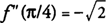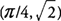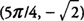## Second Derivative Test for Local Extrema

The second derivative may be used to determine local extrema of a function under certain conditions. If a function has a critical point for which f′(x) = 0 and the second derivative is positive at this point, then f has a local minimum here. If, however, the function has a critical point for which f′(x) = 0 and the second derivative is negative at this point, then f has local maximum here. This technique is called Second Derivative Test for Local Extrema.

Three possible situations could occur that would rule out the use of the Second Derivative Test for Local Extrema:Under any of these conditions, the First Derivative Test would have to be used to determine any local extrema. Another drawback to the Second Derivative Test is that for some functions, the second derivative is difficult or tedious to find. As with the previous situations, revert back to the First Derivative Test to determine any local extrema.

Example 1: Find any local extrema of f(x) = x 4 − 8 x 2 using the Second Derivative Test.

f′(x) = 0 at x = −2, 0, and 2. Because f″(x) = 12 x 2 −16, you find that f″(−2) = 32 > 0, and f has a local minimum at (−2,−16); f″(2) = 32 > 0, and f has local maximum at (0,0); and f″(2) = 32 > 0, and f has a local minimum (2,−16).

Example 2: Find any local extrema of f(x) = sin x + cos x on [0,2π] using the Second Derivative Test.

f′(x) = 0 at x = π/4 and 5π/4. Because f″(x) = −sin x −cos x, you find thatand f has a local maximum at. Also,. and f has a local minimum at.

Back to Top
A18ACD436D5A3997E3DA2573E3FD792A